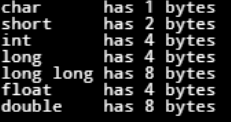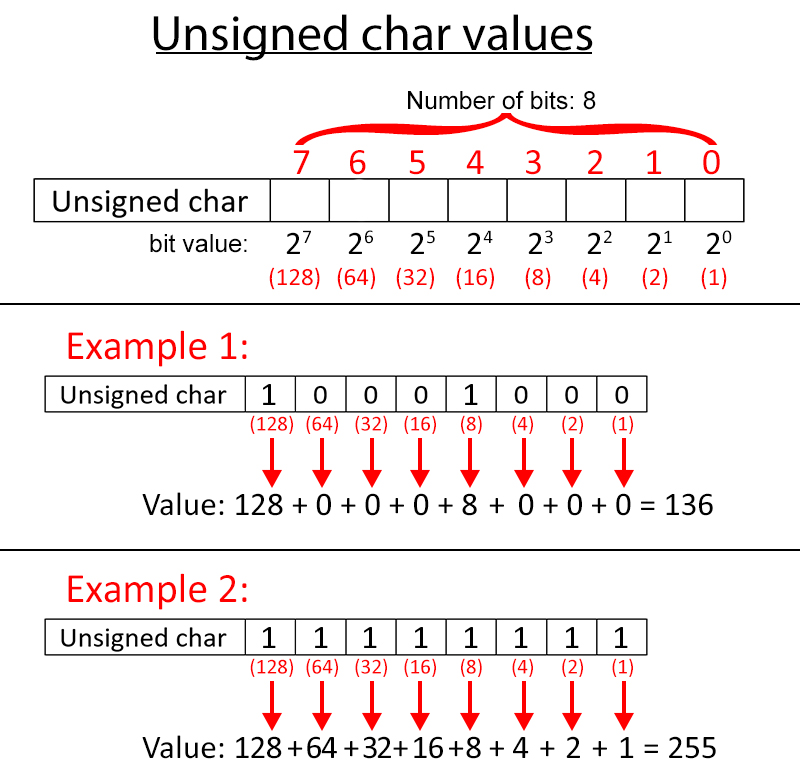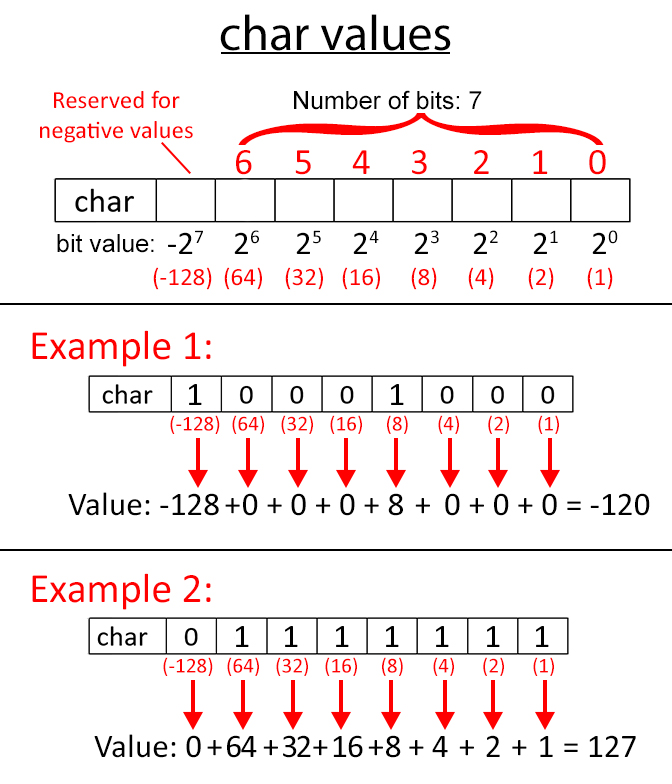# Variables for Integers - Programming

## Types that hold integers: char, short, int, long, long long

Let’s take a look at some examples:

```char cvar = 50;
char cvar = 50;
short svar = 50;
int ivar = 50;
long lvar1 = 50;
long lvar2 = 50;
```

Basic Arithmetic Operators:

Most are familiar with the basic arithmetic operators and the precedence order: + , -, *, /

Here are some examples of how to use the Unary operator: ++ and --:

 Expression Equivalent to Also Equivalent to cvar += 5; cvar = cvar + 5; ++cvar; cvar++; cvar = cvar + 1; --cvar; cvar--; cvar = cvar - 1;
##### Beware of the variable++ vs. ++variable!

++variable

```int x =  10, y = 20;
X = ++y;
```

Note: after this operation: X = 21, and Y also = 21.

variable++

```int x =  10, y = 20;
X = y++;
```

Note: after this operation: X = 20, and Y = 21.

## Compare integer data type

There is a group or data type which allows both positive and negative value – “signed.” However, you do not need to specify that as it is implicit. See below:

 Data type Smallest value Largest value char -2 7 or -128 2 7 - 1 or 127 short -2 15 or -32,768 2 15 - 1 or 32,767 int -2 31 or -2,147,483,648 2 31 – 1 or 2,147,483,647 long -2 31 or -2,147,483,648 2 31 – 1 or 2,147,483,647 long long -2 63 or -9,223,372,036,854,775,808 2 63 – 1 or 9,223,372,036,854,775,807

There is a group or data type which allows only positive value – “unsigned.”

 Data type Smallest value Largest value unsigned char 0 2 8 - 1 or 255 unsigned short 0 2 16 - 1 or 65,535 unsigned int 0 2 32 – 1 or 4,294,967,295 unsigned long 0 2 32 – 1 or 4,294,967,295 unsigned long long 0 2 64 – 1 or 18,446,744,073,709,551,615

## How do you verify the data size?

For the time being, you should know the `sizeof( )` operator. This provides the memory storage unit called “bytes, ” – which will be slightly covered in the next section.

Sample to tell you the # of bytes should type using the operator `sizeof()`. Try this out to verify them yourself. If you change to another controller, you can always do the following to verify.

```Brain.Screen.setFont(fontType::mono30);
Brain.Screen.printAt(1, 20,"char       has %d bytes", sizeof(char));
Brain.Screen.printAt(1, 50,"short      has %d bytes", sizeof(short));
Brain.Screen.printAt(1, 80,"int        has %d bytes", sizeof(int));
Brain.Screen.printAt(1, 110, "long     has %d bytes", sizeof(long));
Brain.Screen.printAt(1, 140, "long long has %d bytes", sizeof(long long));
Brain.Screen.printAt(1, 170, "float     has %d bytes", sizeof(float));
Brain.Screen.printAt(1, 200, "double    has %d bytes", sizeof(double));
```## What does a byte mean?

A “Byte” is a standard unit of data storage.

One Byte = 8 bits. One bit is the smallest memory unit which can only have the value of 1s and 0s (binary value). But, the smallest unit of memory you can acquire from the system is 1 byte; so you cannot ask for 1.5 byte, etc.

Let’s take a look at char. It is short enough to display easily.If it is all filled with 1s, you get 28-1. This value should be the maximum value of “unsigned char” should hold.The system reserves the highest order bit in order to give a negative value. Thus, the maximum value for a “char” ranges from -128 to 127. However, the capacity is still the same as “unsigned char” despite a difference in the data range.

In the computer, it uses an operation called the Two's Complement. This is part of the topics under Bitwise operation, which is out of scope for this document. More on this topic will be discussed in another document.

## What is the difference between char and int?

Here are some common uses for "char" and "int":

```char var1 = ‘9’;
int var2  = 9;
```

It's a common misconception that "char" can only hold symbols such as 'a,' 'b,' or '9.' That's incorrect.

In fact, the "char" type is the same as the "int" type, except that char's capacity is only 1 byte, and int's capacity is 4 bytes. Thus, the data size range is different between the two. You can see that in the data range table above.

The following code segments will give you a clearer view of char and int.

```int iX = '0';
char cX1 = 49;
char cX2 = 50;

Brain.Screen.printAt(3, 20, "int representation of '%c' =  %d",iX, iX);
Brain.Screen.printAt(3, 50, "int representation of '%c' =  %d",cX1, cX1);
Brain.Screen.printAt(3, 80, "int representation of '%c' =  %d",cX2, cX2);
Brain.Screen.printAt(3, 140, "So '%c' - '%c' =  %d ", cX2, iX, cX2 - cX1);
```

Output:

```int representation of ‘0’ = 48
int representation of ‘1’ = 49
int representation of ‘2’ = 50

So ‘2’ – ‘0’ = 1
```

You may wonder: Why ‘0’ shows 48?

This will lead to the topics called “ASCII Code” conversion. ASCII standard is one great IEEE milestones published back in 1963. This is outside the scope of this article. You are encouraged to look up the ASCII Code Table online. You will find a plethora of information online about ASCII Code Table.